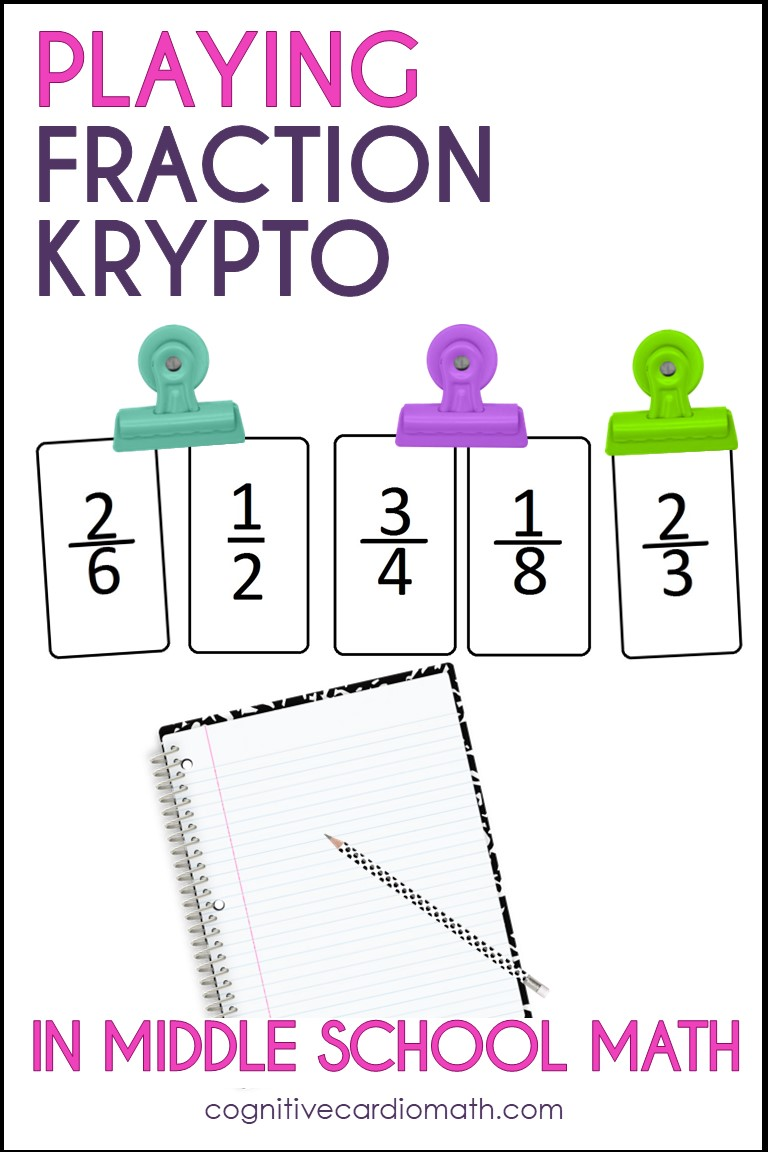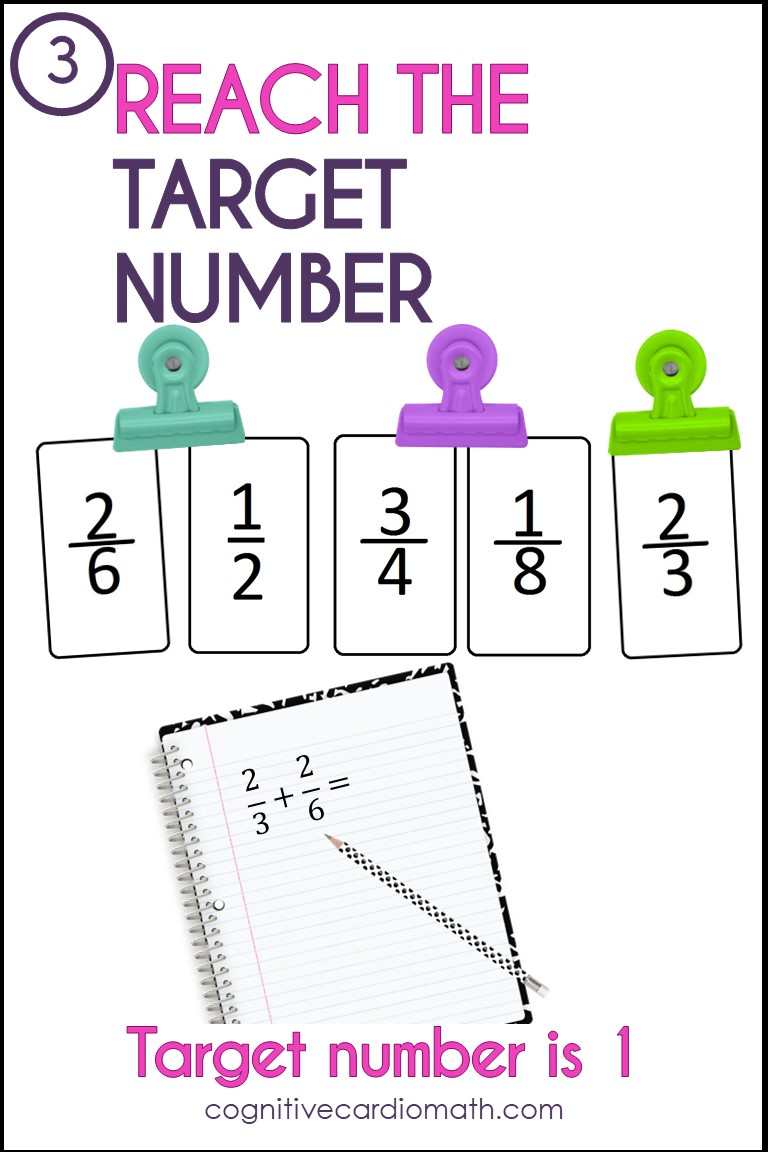# Fraction Review Activity: Fraction KryptoHave you played the math game, Krypto? This fraction activity is fantastic for problem solving, reasoning, and practicing fraction operations skills.
I think your students will love it!

Krypto is an activity I learned about at a conference where Dr. Lola May presented (in like 1993, I think!). I didn’t realize until a long time afterwards that it was a commercial game that could be purchased 🙂   I believe it’s also available as an app now.

​I’ve used the game idea from time to time, following the rules as laid out in the book I got at the conference. Krypto can be played with whole numbers or fractions (and with positive and negative integers as well, I’m sure!).

## Playing Krypto With Fractions

The rules are simple (kind of like the “24” game):
1. Choose 5 common fractions, with denominators of halves, thirds, fourths, sixths, eighths, tenths or twelfths.
2. Students add, subtract, multiply, and/or divide the fractions to make the 5 fractions equal the target number of 1.
3. Students receive points for meeting the target number of 1.

• For example, if they reach the target of 1 using only 3 numbers, they get 300 pts
• 4 numbers = 400 pts
• if they use all 5 numbers, they get 1,000 pts.

You can set up the point system any way you’d like.

Krypto can be used as a team effort/team game or individual enrichment activity.

This math activity is a fantastic way to provide practice with all the fraction operations. It promotes problem solving and persistence.

## Fraction Krypto Example

When I use this activity, I put fraction cards on the board and leave them for as long as I need (and move them if they get in the way!)

• One solution for this set of fractions in the picture could be 3/4 x 2/3 + 1/2 = 1
• This solution uses only 3 fractions, so that would earn 300 points.​ ​
• Another solution is 2/3 + 2/6 = 1; but that’s only 2 fractions. You might consider giving points for using just 2 fractions, until students get the hang of it.

Can you make the 5 fractions equal 1?## Krypto With Whole Numbers

If you’d like to try Krypto with whole numbers, the directions are basically the same:
1) Choose 5 numbers (from 1-20)
2)Choose a target number between 30 and 50).
According to the book I received at the conference (yes, I still have it:-), computers at Berkeley, CA showed that a 5-number solution can be found 86% of the time when the 5 numbers and the target number are chosen randomly.

Example:

• Choose 3, 4, 7, 12, 14
• Target number: 42
• Solution (using 4 numbers): (12 – 4) x 7 – 14 = 42

What other solutions can you find?

If you haven’t used Krypto with either fractions or whole numbers, I hope you’ll give it a try.

Interested in more fraction and fraction operation content?
Check out the fraction program,You can also grab this free fraction operations math wheel (plus other fraction goodies) when you join the email community!

## EllieWelcome to Cognitive Cardio Math! I’m Ellie, a wife, mom, grandma, and dog ‘mom,’ and I’ve spent just about my whole life in school! With nearly 30 years in education, I’ve taught:

• All subject areas in 4th and 5th grades
• Math, ELA, and science in 6th grade (middle school)

I’ve been creating resources for teachers since 2012 and have worked in the elearning industry for about five years as well!